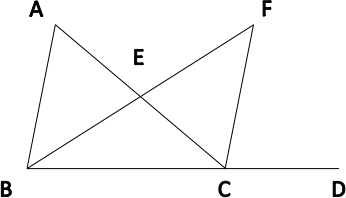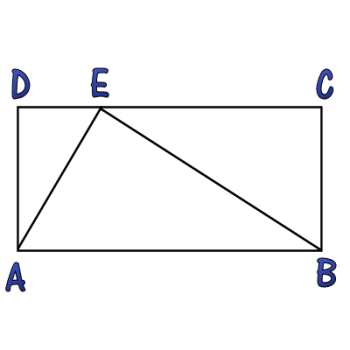Games
Problems
Go Pro!

# Triangle in a Square

Pro Problems > Math > Geometry > Triangles

## Triangle in a Square

Triangle ABC is inside square ABDE, and C lies along side DE. If the area of the triangle is 24 square units, what is the length of AB?

Presentation mode
Problem by allie

## Solution

In order to make it feasible for teachers to use these problems in their classwork, no solutions are publicly visible, so students cannot simply look up the answers. If you would like to view the solutions to these problems, you must have a Virtual Classroom subscription.Assign this problem

## Similar Problems

### Triangle in a Square

A triangle is placed on the interior of a square so that two of the triangle's vertices lie on two adjacent vertices of the square, and the third vertex lies on the opposite side,
1
3
of the way between the two endpoints.

If the perimeter of the triangle (in linear units) is equal to the area of the triangle (in square units), what is the length of the side of the square?

### Three Triangles

The angles in a triangle have measures x, x + 10, and y.

The angles in a second triangle have measures x + y, 40, and 50.

The angles in a third triangle have measures x – y, 40, and z.

What is the value of z?

### Angle Riddle

The angles of a triangle meet the following criteria:

• All the angle measures are prime numbers.
• All the angle measures are distinct.
• Exactly two of my angle measures are palindromic.
• The difference between my two largest angle measures is a two digit number x.
• One of the digits of x is twice the other digit.

### Angle Bisector

In the diagram shown,m ∠DCF = 80
m ∠ACF = 30
m ∠FBD = 50.

If segment BF bisects  ∠ABD, find m ∠BAC.

The angles in a triangle have measures x2 - 5, 2x + 18, and x + 37. What is the measure of the largest angle in the triangle?

### Two Triangles with Angle Measures

In a triangle, the angles measures are: 3x + 44, x, and 2x - 20. In a second triangle, two of the angle measures are x + 20 and 2x - 5. What is the measure of the third angle?

### Triangle and RectangleIn the diagram displayed, the triangle splits the rectangle into three similar triangles. Find the value of AD2 divided by the product of DE and EC.

### Three Two-Digit Angle Measures

The measures of the angles in a triangle are all two digit numbers, and two of them end in 3. Exactly two of them contain the digit 4. None of the angle measures are multiples of three. What are the measures of the angles?

### Angles in a Triangle

In a triangle, the measure of the largest angle is 12 less than the sum of the measures of the other two angles. The largest angle is also 52 more than the twice the middle angle decreased by three times the smallest angle. What is the largest angle measure?

### Arithmetic Angles

The angles of a triangle are in arithmetic progression, and the difference between the largest and smallest angle is 42 degrees. What are the three angles?

Forty-five and Thirty, Split Triangle, Triangle Variables, Angle MeasureLike us on Facebook to get updates about new resources